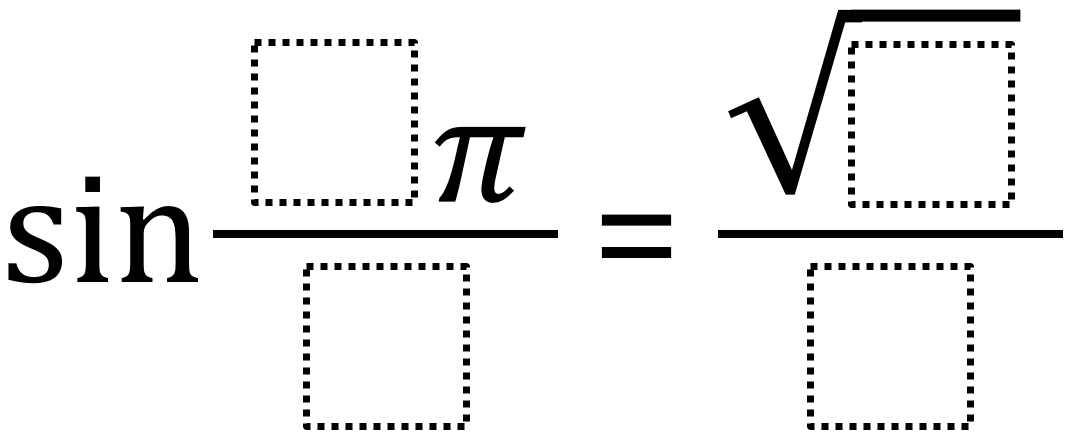Home > High School: Functions > Sine Functions 2

# Sine Functions 2

Directions: Using the digits 1 to 9 at most one time each, place a digit in each box to find the function’s greatest possible value.### Hint

What values of pi are more likely to result in a larger value?
How can equivalent fractions allow us to avoid using a number more than once?

Crystal Spellmann found the greatest possible value of 1 by using sin 3π/6=√4/2

Source: Robert Kaplinsky

## Creating Sequences

Directions: Using the digits 1-9, at most one time each, complete the first three terms …

1.Crystal Spellmann

I used sin 3π/6=√4/2. The largest value for the sine function is 1.

•Robert Kaplinsky

Thanks Crystal! I’ll get this corrected.

2.Other solutions: sin(1π/2)=√9/3, sin(2π/4)=√9/3. New problem idea using digits 1-9 each at most once (not sure if its here already: sin ( (_)pi/(_)) + (cos (_)pi/(_)) = sqrt()/()